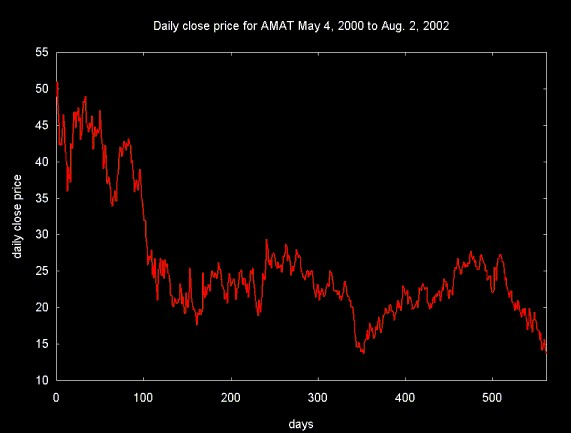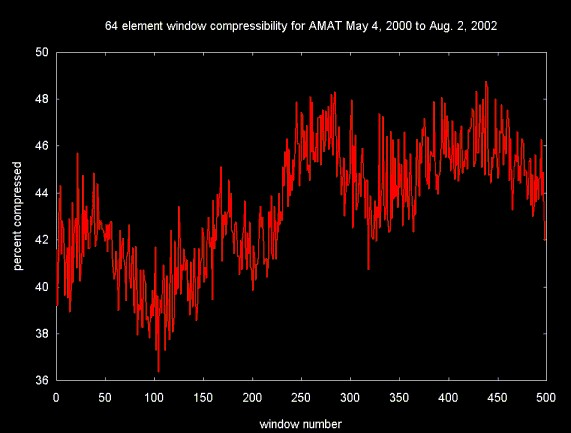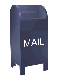# Wavelet compression, determinism and time series forecasting

We do not want to merely recognize patterns; we want to find the patterns that are there to be recognized. We do not only want to forecast; we want to know the hidden mechanisms that make the forecasts work. The point, in other words, is to find the causal, dynamical structure intrinsic to the process we are investigating, ideally to extract all the patterns in it that have any predictive power.

An Algorithm for Pattern Discovery in Time Series by Cosma Rohilla Shalizi, Kristina Liza Shalizi and James P. Crutchfield, Santa Fe Institute Working Paper 02-10-060, November 2002

# Under Construction

### Abstract

This web page examines lossless wavelet compression as a tool for time series forecasting. Several simple time series "predictors" are developed. These predictors are applied to synthetic random time series and to stock market time series. The amount of noise (or non-determinism) is examined using wavelet compression and several other techniques, for the purposes of comparison. Various simple statistical tools are used for analyzing the predictors and the time series, including correlation and the autocorrelation function.

### Forecasting the Future

Humans have always wanted to forecast the future. In caves lit by tallow lamps whose flicker lit paintings of bison and antelope, shamans threw marked bones to foretell the future. The Greeks of classical history consulted oracles, the most famous being the oracle at Delphi.

The prehistoric shaman asked whether the hunt would succeed or asked where the tribe should travel to find the animal herds. The Greek oracles were asked if an endevor would succeed or what course of action should be followed. These are all questions involving long term forecasting. Long term forecasts have the most potential profit, but also high uncertainty. As the accuracy of modern economic forecasts demonstrates, long term forecasting is fraught with error. This web page deals with short term forecasting. Less profit exists in short term forecasts, but there is less error and there is some hope of quantifying this error.

Within a limited scope, modern computers, mathematics and algorithms give forecasting an empirical foundation. Successful short term forecasting is based on the statistical properties of a data set. If an underlying deterministic process generated the time series that we are attempting to forecast, a forecasting technique may have some hope of success. In practice the deterministic process may be very complex (e.g., a market). The future is always unknown and to some extent, unknowable. Forecasting is always imperfect, but this is not the same as useless.

A proposed forecasting technique can be tested on a subset of past data (an "in-sample" test) and retested on an "out-of-sample" subset. Rigorous testing on past data sets provides some indication of how reliable the forecasting technique may be when used to infer future values from past values.

### Time Series and Determinism

A time series is a data set where each data element sk is associated with a time element tk. Examples of time series include the water level of the Nile river in Egypt, sun spots, the stock market close price for IBM, a digital recording of a Jazz pianist playing at a night club (in this case the time component of the time series is determined by the sampling rate) and the neutron emission of a radioactive element as the element decays.

Radioactive decay is an entirely random process and quantum mechanics states that it is unpredictable (as Schrödinger's cat can attest). The other time series I have listed above all result from some underlying deterministic process. For example, seasonal flooding of the Nile river, the action of buyers and sellers in a stock market or a Jazz scale. The determinism in these time series may be intermixed with non-deterministic data (e.g., noise).

There is a sizable literature on time series forecasting and a variety of sophisticated techniques have been applied to this problem. The success of any forecasting technique depends on the presence of an underlying deterministic process that generated the time series. No matter how powerful the forecasting technique is, a random process like radioactive decay cannot be predicted. Although the exact day of the month that the Nile flood starts cannot be predicted, the fact that the river level rose yesterday makes it more likely to rise today.

### Wavelet Compression

The trade-off between return and risk plays a prominent role in many financial theories and models, such as Modern Portfolio theory and option pricing. Given that volatility is often regarded as a measure of this risk, one is interested not only in obtaining accurate forecasts of returns on financial assets, but also in forecasts of the associated volatility. Much recent evidence shows that volatility of financial assets is not constant, but rather that relatively volatile periods alternate with more tranquil ones. Thus, there may be opportunities to obtain forecasts of this time-varying risk.

From the Introduction in Non-linear time series models in empirical finance by Philip Hans Franses and Dick van Dijk, Cambridge University Press, 2000

Wavelet compression provides one measure for estimating the amount of determinism in a time series. If we have a wavelet function that approximates a data set, then data that cannot be approximated is non-deterministic, relative to the approximation function (for a related discussion see Predict Wavelets: wavelets viewed as compression and Lossless Wavelet Compression). If a wavelet approximation function is matched with the forecasting function, the wavelet estimation of determinism provides an indication of how accurate the result of the forecasting function may be.

If there is a long time series, it can be broken up into sections, each of which has a power of two number of elements. Wavelet compression can be applied to each of these sections, yielding a single number: the amount of compression for that section. These compression values form a derived time series, where each compression value is associated with a time window. A region where there is low compressibility is also likely to be a region where the success of the forecasting technique is low. If a forecast value is calculated from a data set in a relatively incompressible region it can be discarded or discounted by some factor.

Figure 1 below shows the close price for Applied Materials (AMAT) over 563 trading days (about two years and a quarter).

Figure 1Data from finance.yahoo.com

To generate Figure 2 a sliding window was advanced one element at at time through the data in Figure 1. The percentage of compression using lossless wavelet compression was estimated for each window and plotted on the y-axis. The window number is plotted on the x-axis. It is interesting to note that compressibility varies over time (from slightly more than 38% to a little over 48%).

Figure 2Several other metrics will be examined below to provide a comparison for wavelet compression.

### Predictors

This discussion of forecasting focuses on short term forecasting, were there is at least an assumed underlying deterministic process that generated the time series (e.g., the time series is not random). Although there is always more profit in long term forecasting, from a mathematical point of view, there is more reliability in short term forecasting.

Forecasting relies on a predictive function (a predictor) which uses a known part of a time series to generate a future prediction. The amount of noise (non-determinism) that is present in the known time series region that is used to generate the forecast value will have some influence on how close a forecast value is to the actual future value. Wavelet compression provides one way to estimate the amount of noise (or non-determinism) in a time series region. If the forecast (predictor) is calculated on a high noise region, the forecast may be less reliable than a forecast drawn from a low noise (more deterministic) region.

Several predictors for financial time series are derived and discussed below. These predictors are used along with noise estimation techniques to evaluate the usefulness of wavelet compression in a forecasting setting.

### Denoising and Noise Models

If the noise in a time series region can be estimated, why not just remove the noise? A forecast can then be calculated on a data set from the denoised time series. This forecast may be more reliable, since the noise has been removed.

Noise can be removed from a time series using a variety of techniques. For example, simple low pass filters, like a moving average can be used to remove the high frequency component of a time series. This can be useful if the time series contains high frequency noise. A variety of more sophisticated high and low pass filters can be constructed using wavelet or Fourier transforms. Wavelet thresholding can be used to remove components of the time series based on a particular noise model (e.g., Gaussian noise).

The danger in applying these filters is that important information may be removed along with the those components that are labeled noise. Techniques like Gaussian noise removal assume a particular noise model. If this model does not fit the noise present in the time series, the filter may not uncover the underlying signal.

Denoising techniques that rely on wavelet compression are not based on assumptions about the underlying noise. The core assumption is that the wavelet function provides an approximation at various scales for the data set. The success (or failure) of the technique relies on information theoretic issues. Denoising using compression closely parallels the technique I've outlined here. There may be cases when denoising the forecast data before calculating the forecast value is preferable to calculating both the forecast (on a noisy data set) and a reliability estimate (using wavelet compression).

### Software and Documentation

The work that went into these web pages has been a long time in the making. This work is still not done, so these web pages remain "under construction". I was hoping to escape the Silicon Valley rat race into a stable environment where I could spend my spare time working on quantitative finance. Sadly, things did not work out as I hoped. I need to return to my previous love, optimizing compilers, since an optimizing compiler has more commercial promise. So I am not sure when this work will be finished. I believe what I have outlined here is promising, so I am publishing the preliminary software. I have not done the usual build testing, so it is not up to my usual standards. Much still remains to be done and I still hope to finish this work. I hope that the interim software may still be useful to others.

• Lossless wavelet compression (and other wavelet packet) software, in UNIX tar/gzip format: compress.tar.gz

### References (books)

• Ripples in Mathematics by Jensen and la Cour-Harbo, Springer Verlag, 2001

This book has been the primary reference I have used to develop the wavelet packet algorithms that are used to calculate the minimum data set (and compressibility) for a time series.

• Wavelet Methods for Time Series Analysis by Percival and Walden, Cambridge University Press, 2000

Wavelet Methods for Time Series Analysis is a much more mathematically intensive text on wavelets and wavelet methods. Examples of the application of wavelet techniques to a number of time series are covered in this book, including sun spots and the Nile river flood.

• Noise Reduction by Wavelet Thresholding, Maarten Jansen, Springer Verlag Lecture Notes in Statistics (161), 2001

This book provides a detailed discussion of noise removal using a variety of wavelet thresholding techniques. Background material on the wavelet Lifting Scheme is also covered (although not as clearly as in Ripples in Mathematics.

• Applied Bayesian Forecasting and Time Series Analysis by Pole, West and Harrison, Chapman and Hall, CRC Press, 1999

This text covers a variety of time series forecasting techniques and includes examples in a variety of different areas.

• Chaos and Fractals: New Frountiers of Science by Peitgen, Jürgens and Saupe, Springer-Verlag, 1992

There are several areas where wavelets and fractals intersect. For example, the Daubechies wavelets are fractals. Fractal wavelets have excellent filtering characteristics in some cases. This fantastic book by Peitgen et al not only provides a readable introduction to wavelet theory, it covers issues about noise and estimation of determinism in a data set. This book is a classic and anyone who enjoys computer science and/or mathematics will love this book.

• The Cartoon Guide To Statistics by Larry Gonic and Woollcott Smith, Harper Collins

Although the title is a bit embarrassing, The Cartoon Guide to Statistics provided a very rapid and readable introduction to statistics. It remains one of the best references I have for basic statistics.

### Wavelet references on the Web

• Ripples in Mathematics web page.

This is the web page by the authors of Ripples in Mathematics. It includes links to a number of sub-pages, including pages that publish Matlab and C source code for the algorithms discussed in the book.

### Some quantitative finance references on the Web

Ian Kaplan
May 2003
Revised: